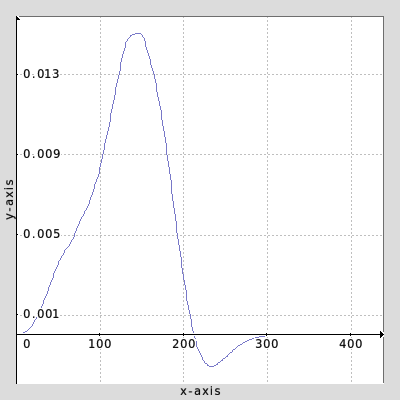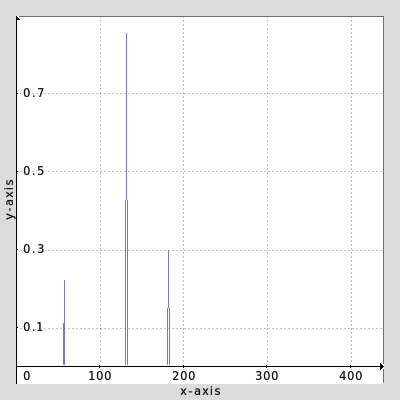# Some linear solver stuff in g'mic

I’ve been working on a non-negative least squares solver command, based on FNNLS algorithm. Although it does work, it has what I suspect is a precision-related problem - there are certain inputs which never converge, i.e. it loops forever. It’s also possibly just a straightforward script error.

I’m posting it here, because I’m about to run out of time on it for now and I don’t want to push a command which can infinite loop. The hope is somebody else might find a fix, or rewrite it!

``````# Non-negative least squares
# Solve Ax = b, xi>0 for selected A matrix and specified b vector
gcd_solve_nonnegative : check \${"is_image_arg \$1"}
pass\$1 0 => B
repeat \$!-1 { l[\$>,-1] {
M,N:=[h#0,w#0] act=\$N
# Active and passive indexes are held in a single vector: [ind]
1,\$N,1,1 => X . => S . => W +f. y => ind
+transpose . mmul..  mmul. [B] =>.. AtA => AtB

for \$act>0 {
# W = AtB - AtA*x (negative gradient)
j[W] [AtB] +mmul[AtA] [X] sub[W,-1]
1,\$act,1,1,"i[#\$W,i[#\$ind,y]]" => Wact

# EXIT if max (negative) gradient is close to zero
if iM<1e-6 rm[Wact] break fi

# Move index of max Wact element from active to passive list
eval "
a_index = yM;
w_index = i[#\$ind,a_index];
copy(i[#\$ind,a_index],i[#\$ind,a_index+1],\$N-1-a_index);
i[#\$ind,\$N-1] = w_index;"
rm[Wact] act:=\$act-1

# Enforce non-negative constraint
for 1 {
# Solve As = b for current passive set
part:=\$N-\$act
sh[ind] \$act,100%,0,0 => psv # passive indexes
\$part,\$part,1,1,"i(#\$AtA,i[#\$psv,x],i[#\$psv,y])" => Apsv
1,\$part,1,1,"i[#\$AtB,i[#\$psv,y]]" => Bpsv
+solve[Bpsv] [Apsv] => Spsv

# Copy passive solution to S, set to zero for active variables
eval[S] "i[i[#\$ind,y]]=y<\$act?0:i[#\$Spsv,y-\$act]"
rm[Apsv,Bpsv]

# EXIT moving negatives if passive solution is all positive
if im>0 rm[psv,Spsv] break fi

# Calculate possible scaling ratios
1,\$part,1,1,"i[#\$X,i[#\$psv,y]]" => Xpsv
1,\$part,1,1,"XP=i[#\$Xpsv,y];XP/(XP-i[#\$Spsv,y])" => ratio

# Find minimum ratio
eval[Spsv] "
begin(MR=inf);
MR=i>0?MR:min(MR,i[#\$ratio,y]);
end(set('alpha',MR))"
rm[Xpsv,ratio]

# X = X + alpha*(S-X) (Do the scaling)

# Move indexes from passive to active where X[p_index] <= 0
eval[psv] "
begin(t=0);
p_index = i;
if(i[#\$X,p_index]<=0,
copy(i[#\$ind,1],i[#\$ind,0],y+\$act);
i[#\$ind,0] = p_index;
t++;
);
end(set('act',\$act+t))"
rm[psv,Spsv]
}
j[X] [S]
}
k[X]
} }
``````

Example usage:
`gmic 4,3,1,1,"round(u(10))" 1,3,1,1,"round(u(10))" +gcd_solve_nonnegative.. .`

Example failure inputs (never ends):
`gmic "(-250517.890625,224689,315384.71875,-272708.90625;-239055.84375,60966.41015625,174796.484375,-51424.0234375;63545.421875,-52172.0234375,-76025.2421875,62647.45703125)" "(1;1;1)" +gcd_solve_nonnegative.. .`

The main differences to the usual FNNLS are:

• This uses a different loop format using `break` to avoid duplicated code. That’s normally considered bad (but this is my choice)
• The active and passive indexes are held in a single image/vector. I don’t know if that’s a good method.
2 Likes

Update: not certain if the below exits for all cases, but leaving here for reference.

I may have a solution: instead of checking the most negative gradient is low enough, compare it to the previous gradient. If it hasn’t improved then we’re done. Initialise with `best:=inf`, then:

``````      # EXIT if most negative gradient hasn't improved
new:=iM
if \$new<1e-6" || "\$new>=\$best rm[Wact] break fi
best=\$new
``````

replaces the old version:

``````      # EXIT if max (negative) gradient is close to zero
if iM<1e-6 rm[Wact] break fi
``````

Update 2:

The above can still get stuck in the non-negative constraint part. If the exit criteria there is changed to greater than or equal to zero, then the problem disappears. I’m not certain whether this has an effect on getting a more optimal solution, but will have to do for now:

``````# EXIT moving negatives if passive solution is all non-negative
if im>=0 rm[psv,Spsv] break fi
``````
1 Like

I wish I had a visual explanation of how that works so that I can assist. Most of my learning of other things comes through visual explanation. Best of luck to you.

Well, it seems the convergence is fixed with the above two changes. I’ll finish up the command and push it soon.

I know exactly what you mean about visuals - just having a picture of the matrices in my head helps me a lot, but there’s a geometric way of viewing what’s happening too. I don’t know if I’ll have the time to produce such a thing though.

1 Like

Sadly I found more cases where it hangs. I’ll probably need to check some other implementations, but any help would still be good1 Like

Draw it on tissue paper. Does not have to look nice.1 Like

Now I think I’ve finally solved it, will do more tests and push soon. The part which scales vector x according to a min ratio should always set the associated entry in x to zero:

`x + a(s-x) = x + (x/(x-s))(s-x) = x - x = 0`

Probably what happens is this doesn’t quite cancel out, so the simple fix is to just set that single entry to zero after the scaling. I think that also means I can remove one of the other fixes doing `im>0` as it should be.

2 Likes

What would be the application of this?

Anywhere that you need a “best fit” solution, but you know it should never be negative. A silly example which also shows limitations (there are better ways):

Say you want to find a spectral power distribution for a light L which produces xyz coords of (0.5,1,0.5). You might just solve it with `M'x = L`, where M is an n*3 matrix of xyz colour matching functions. Unfortunately, you get “negative energy” at some wavelengths, not physically possibleInstead, you can do the same solving with non-negative to get this:You get several narrow wavelength bands, but at least they’re all positive.

2 Likes

One I’ve had sitting around for a while which I might finish and push soon: canonical correlation

``````# selections: two matrices with dims: n*p1, n*p2, p1<=p2
# outputs: correlations, A, B
gcd_cancorr :
l[-2,-1] {
D:=min(w#0,w#1)-1
# u1,s1,v1 u2,s2,v2
foreach { +r 100%,1,100%,100%,2 - / {sqrt(h-1)} svd }
transpose mmul[0,3] # u1'u2
svd # U,S,V
=> U => S => V
=> s1 => v1 => s2 => v2
z[U,V] 0,0,\$D,100%
pow[s1,s2] -1 diagonal[s1,s2] # s1` s2`
mmul[v1] [s1] mmul[v1] [U] =>[v1] a # a = v1s1`U
mmul[v2] [s2] mmul[v2] [V] =>[v2] b # a = v2s2`V
k[S,a,b]
}
``````
2 Likes

And another which I’ll probably expand with more options (e.g. specify one factor): non-negative factors

``````# non-negative matrix factorisation
# selection: matrix to factor
# inputs: width, iters
gcd_factor_nonnegative : skip \${1=1},\${2=100}
foreach {
M,N,X:=[h,w,max(\$1,1)]
+r \$X,\$M,1,1,2 => W
+r \$N,\$X,1,1,2 => H
repeat \$2 {
# H = H * (W' / W'WH)
+transpose[W] => Wt
[Wt] mmul. [W] mmul. [H] # W'WH
+mmul[Wt]  rm[Wt]
*[H,-1] +eq. 0 +[-2,-1] /[H,-1]
# W = W * (H' / WHH')
+transpose[H] => Ht
+mmul[W] [H] mmul. [Ht]
+mmul [Ht] rm[Ht]
*[W,-1] +eq. 0 +[-2,-1] /[W,-1]
}
k[W,H]
}
``````
2 Likes

Nice! Crush those negatives with impunity!

Good to see you active on the forum again.1 Like